Next: 13.2 Cylinders
Up: 13 Polyhedra
Previous: 13 Polyhedra

# 13.1 Regular Polyhedra

Figure 1 shows the five regular polyhedra, or Platonic solids.Figure 1: The Platonic solids. Top: the tetrahedron (self-dual). Middle: the cube and the octahedron (dual to one another). Bottom: the dodecahedron and the icosahedron (dual to one another).

In the following tables and formulas, a is the length of an edge,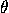the dihedral angle at each edge, R the radius of the circumscribed sphere, r the radius of the inscribed sphere, V the volume, S the total area, v the total number of vertices, e the total number of edges, f the total number of faces, p the number of edges in a face, and q the number of edges meeting at a vertex.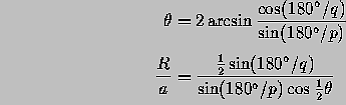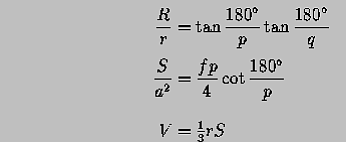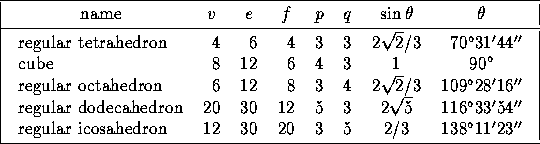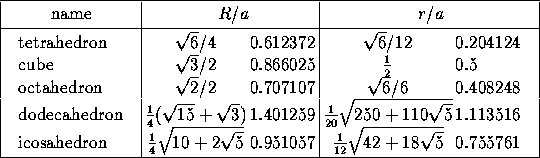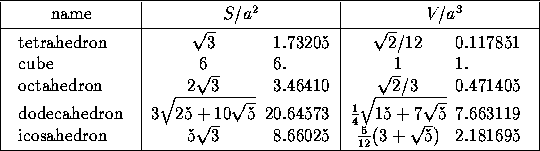Next: 13.2 Cylinders
Up: 13 Polyhedra
Previous: 13 PolyhedraThe Geometry Center Home Page

Silvio Levy
Wed Oct 4 16:41:25 PDT 1995

This document is excerpted from the 30th Edition of the CRC Standard Mathematical Tables and Formulas (CRC Press). Unauthorized duplication is forbidden.